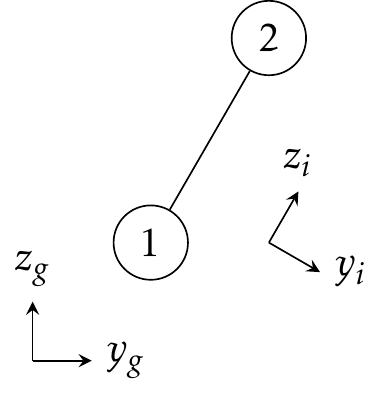# Quasi-Internal Frame¶Fig. 2 Global frame g and QI frame i of atoms 1 and 2. The z direction of this QI frame is chosen along the distance vector.

## Rotation Matrix¶

Consider two vectors u, v and two reference frames A, B. R is the rotation matrix of the axes such that

\begin{align}\begin{aligned}R u_A = u_B,\\R v_A = v_B.\end{aligned}\end{align}

Since $$u_A^T v_A=u_B^T v_B$$,

$R^T R=I.$

A 2-D tensor, e.g., quadrupole moment Q, in two reference frames are associated by

$u_A^T Q_A v_A = u_B^T Q_B v_B.$

It is easy to prove that

$R Q_A R^T = Q_B.$

Two common transformations used in Tinker are:

• From (A) Local Frame (in which parameters are provided) to (B) Global Frame (in which the calculation is done);

• From (A) Global Frame (for direct pairwise electrostatics) to (B) Quasi-Internal (QI) Frame (for optimized algebra), as shown in Fig. 2.

## Multipole Interaction in QI Frame¶

Once the distance vector is in QI frame, many derivatives can be simplified as shown in the following table. f(r) does not explicitly depend on $$r_x,r_y,r_z$$.

Global Frame

QI Frame

$$\partial f(r)/\partial x_2$$

$$f'(r)r_x/r$$

0

$$\partial f(r)/\partial y_2$$

$$f'(r)r_y/r$$

0

$$\partial f(r)/\partial z_2$$

$$f'(r)r_z/r$$

$$f'(r)$$

For potential energy, (4) can be used without modification in QI frame. Since $$\partial\phi_1/\partial z_1 = -E_{z1}$$, the z direction gradient can be obtained from z direction electrostatic field (Ez):

$\frac{\partial U}{\partial z}=-E_z C -E'_z D -E''_z Q -\cdots.$

Once the torques are computed the same way as in the previous section

$\tau = \tau_1 + \tau_2 = \boldsymbol{r}\times\boldsymbol{F} = (U'_x,U'_y,U'_z)\times(0,0,r) = (U'_y r, -U'_x r, 0),$

x and y direction gradients then become

$\begin{split}U'_x &= -\tau_y/r, \\ U'_y &= \tau_x/r.\end{split}$

Depending on the direction of distance vector, the signs of x and y direction gradients may flip.

## Details¶

In the following notes, $$A : B$$ stands for $$A = A + B$$. If there is no ambiguity, $$f'$$ and $$f''$$ may stand for $$(f'_x,f'_y,f'_z)$$ and $$(f''_{xx},f''_{yy},f''_{zz},f''_{xy},f''_{xz},f''_{yz})$$, respectively.

Potential Terms

Notes

$$\phi_1$$

$$\phi_1$$

$$\phi'_{1x}$$

$$\partial\phi_1/\partial x_1$$

$$\phi'_{1y}$$

$$\partial\phi_1/\partial y_1$$

$$\phi'_{1z}$$

$$\partial\phi_1/\partial z_1$$

$$\phi''_{1xx}$$

$$\partial^2\phi_1/\partial x_1^2$$

$$\phi''_{1yy}$$

$$\partial^2\phi_1/\partial y_1^2$$

$$\phi''_{1zz}$$

$$\partial^2\phi_1/\partial z_1^2$$

$$\phi''_{1xy}$$

$$\partial^2\phi_1/\partial x_1\partial y_1$$

$$\phi''_{1xz}$$

$$\partial^2\phi_1/\partial x_1\partial z_1$$

$$\phi''_{1yz}$$

$$\partial^2\phi_1/\partial y_1\partial z_1$$

$$\phi_2$$

$$\phi_2$$

$$\phi'_{2x}$$

$$\partial\phi_2/\partial x_2$$

$$\phi'_{2y}$$

$$\partial\phi_2/\partial y_2$$

$$\phi'_{2z}$$

$$\partial\phi_2/\partial z_2$$

$$\phi''_{2xx}$$

$$\partial^2\phi_2/\partial x_2^2$$

$$\phi''_{2yy}$$

$$\partial^2\phi_2/\partial y_2^2$$

$$\phi''_{2zz}$$

$$\partial^2\phi_2/\partial z_2^2$$

$$\phi''_{2xy}$$

$$\partial^2\phi_2/\partial x_2\partial y_2$$

$$\phi''_{2xz}$$

$$\partial^2\phi_2/\partial x_2\partial z_2$$

$$\phi''_{2yz}$$

$$\partial^2\phi_2/\partial y_2\partial z_2$$

### Charge Terms¶

$\begin{split}\phi_1 &: T_{12}^{(1,1)} C_2 = B_0 C_2,\ \phi'_1 : T_{12}^{(2:4,1)} C_2 = \begin{pmatrix} 0 \\ 0 \\ r B_1 C_2 \end{pmatrix}, \\ \phi''_1 &: T_{12}^{(5:13,1)} C_2 = -\begin{pmatrix} B_1 C_2 \\ B_1 C_2 \\ (B_1 - r^2 B_2) C_2 \\ 0 \\ 0 \\ 0 \end{pmatrix}.\end{split}$
$\begin{split}\phi_2 &: T_{21}^{(1,1)} C_1 = B_0 C_1,\ \phi'_2 : T_{21}^{(2:4,1)} C_1 = -\begin{pmatrix} 0 \\ 0 \\ r B_1 C_1 \end{pmatrix}, \\ \phi''_2 &: T_{21}^{(5:13,1)} C_1 = -\begin{pmatrix} B_1 C_1 \\ B_1 C_1 \\ (B_1 - r^2 B_2) C_1 \\ 0 \\ 0 \\ 0 \end{pmatrix}.\end{split}$
$\begin{split}-E_{z1} &: r B_1 C_2,\ -E'_{z1} : -\begin{pmatrix} 0 \\ 0 \\ B_1 - r^2 B_2 \end{pmatrix}, \\ -E''_{z1} &: -\begin{pmatrix} r B_2 C_2 \\ r B_2 C_2 \\ (3 r B_2 - r^3 B_3) C_2 \\ 0 \\ 0 \\ 0 \end{pmatrix}.\end{split}$

### Dipole Terms¶

$\begin{split}\phi_1 &: T_{12}^{(1,2:4)} D_2 = -r B_1 D_{z2},\ \phi'_1 : T_{12}^{(2:4,2:4)} D_2 = \begin{pmatrix} B_1 D_{x2} \\ B_1 D_{y2} \\ (B_1 - r^2 B_2) D_{z2} \end{pmatrix}, \\ \phi''_1 &: T_{12}^{(5:13,2:4)} D_2 = \begin{pmatrix} r B_2 D_{z2} \\ r B_2 D_{z2} \\ (3 r B_2 - r^3 B_3) D_{z2} \\ 0 \\ 2 r B_2 D_{x2} \\ 2 r B_2 D_{y2} \end{pmatrix}.\end{split}$
$\begin{split}\phi_2 &: T_{21}^{(1,2:4)} D_1 = r B_1 D_{z1},\ \phi'_2 : T_{21}^{(2:4,2:4)} D_1 = \begin{pmatrix} B_1 D_{x1} \\ B_1 D_{y1} \\ (B_1 - r^2 B_2) D_{z1} \end{pmatrix}, \\ \phi''_2 &: T_{21}^{(5:13,2:4)} D_1 = -\begin{pmatrix} r B_2 D_{z1} \\ r B_2 D_{z1} \\ (3 r B_2 - r^3 B_3) D_{z1} \\ 0 \\ 2 r B_2 D_{x1} \\ 2 r B_2 D_{y1} \end{pmatrix}.\end{split}$
$\begin{split}-E_{z1} &: (B_1 - r^2 B_2) D_{z2},\ -E'_{z1} : \begin{pmatrix} r B_2 D_{x2} \\ r B_2 D_{y2} \\ (3 r B_2 - r^3 B_3) D_{z2} \end{pmatrix}, \\ -E''_{z1} &: -\begin{pmatrix} (B_2 - r^2 B_3) D_{z2} \\ (B_2 - r^2 B_3) D_{z2} \\ (3 B_2 - 6 r^2 B_3 + r^4 B_4) D_{z2} \\ 0 \\ 2 (B_2 - r^2 B_3) D_{x2} \\ 2 (B_2 - r^2 B_3) D_{y2} \end{pmatrix}.\end{split}$

$\begin{split}\phi_1 &: T_{12}^{(1,5:13)} Q_2 = r^2 B_2 Q_{zz2},\ \phi'_1 : T_{12}^{(2:4,5:13)} Q_2 = -\begin{pmatrix} 2 r B_2 Q_{xz2} \\ 2 r B_2 Q_{yz2} \\ (2 r B_2 - r^3 B_3) Q_{zz2} \end{pmatrix}, \\ \phi''_1 &: T_{12}^{(5:13,5:13)} Q_2 = \begin{pmatrix} 2 B_2 Q_{xx2} - r^2 B_3 Q_{zz2} \\ 2 B_2 Q_{yy2} - r^2 B_3 Q_{zz2} \\ (2 B_2 - 5 r^2 B_3 + r^4 B_4) Q_{zz2} \\ 4 B_2 Q_{xy2} \\ 4 (B_2 - r^2 B_3) Q_{xz2} \\ 4 (B_2 - r^2 B_3) Q_{yz2} \end{pmatrix}.\end{split}$
$\begin{split}\phi_2 &: T_{21}^{(1,5:13)} Q_1 = r^2 B_2 Q_{zz1},\ \phi'_2 : T_{21}^{(2:4,5:13)} Q_1 = \begin{pmatrix} 2 r B_2 Q_{xz1} \\ 2 r B_2 Q_{yz1} \\ (2 r B_2 - r^3 B_3) Q_{zz1} \end{pmatrix}, \\ \phi''_2 &: T_{21}^{(5:13,5:13)} Q_1 = \begin{pmatrix} 2 B_2 Q_{xx1} - r^2 B_3 Q_{zz1} \\ 2 B_2 Q_{yy1} - r^2 B_3 Q_{zz1} \\ (2 B_2 - 5 r^2 B_3 + r^4 B_4) Q_{zz1} \\ 4 B_2 Q_{xy1} \\ 4 (B_2 - r^2 B_3) Q_{xz1} \\ 4 (B_2 - r^2 B_3) Q_{yz1} \end{pmatrix}.\end{split}$
$\begin{split}-E_{z1} &: -(2 r B_2 - r^3 B_3) Q_{zz2},\ -E'_{z1} : \begin{pmatrix} 2 (B_2 - r^2 B_3) Q_{xz2} \\ 2 (B_2 - r^2 B_3) Q_{yz2} \\ (2 B_2 - 5 r^2 B_3 + r^4 B_4) Q_{zz2} \end{pmatrix}, \\ -E''_{z1} &: \begin{pmatrix} -2 r B_3 Q_{yy2} - r^3 B_4 Q_{zz2} \\ -2 r B_3 Q_{xx2} - r^3 B_4 Q_{zz2} \\ (12 r B_3 - 9 r^3 B_4 + r^5 B_5) Q_{zz2} \\ 4 r B_3 Q_{xy2} \\ 4 (3 r B_3 - r^3 B_4) Q_{xz2} \\ 4 (3 r B_3 - r^3 B_4) Q_{yz2} \end{pmatrix}.\end{split}$